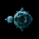1057 obejrzeń
1057
Standard Error Bands - Code by @XeL_arjona
Original implementation by:
Traders issue: Stocks & Commodities V. 14:9 (375-379):
Standard Error Bands by Jon Andersen
Version 1

For a quick and publicly open explanation of this Statistical indicator, you can refer at Here!

Extract from the former URL:
Standard Error bands are quite different than Bollinger's. First, they are bands constructed around a linear regression curve. Second, the bands are based on two standard errors above and below this regression line. The error bands measure the standard error of the estimate around the linear regression line. Therefore, as a price series follows the course of the regression line the bands will narrow, showing little error in the estimate. As the market gets noisy and random, the error will be greater resulting in wider bands.

```// Standard Error Bands - Code by @XeL_arjona
// Original implementation by:
//     Traders issue: Stocks & Commodities V. 14:9 (375-379):
//                    Standard Error Bands by Jon Andersen
// Ver 1
study(title="Standard Error Bands by @XeL_arjona", shorttitle="StDeBands", overlay=true)
len = input(defval=21, minval=1, title="Linear Regression Window:")
sm = input(true, title="Use Jon Andersen's Smooth of Median:")
src = close
// Standard Error Band Function
stdeB(array,p,mult,dir) =>
lr = sm ? sma(linreg(array,p,0),1) : linreg(array,p,0)
stde = stdev(lr,p)/sqrt(p)
d = dir ? 1 : -1
eband = lr + d * mult * stde
lrc = sma(linreg(src, len, 0),1)
m = plot(lrc, color = blue, title = "OLS Regression Curve", style = line, linewidth = 2)
ub = plot(stdeB(close,len,2,true), color = green, title = 'StdEu', style = line, linewidth = 1)
bb = plot(stdeB(close,len,2,false), color = red, title = 'StdEb', style = line, linewidth = 1)
fill(m,ub, color=olive, title="StdE_U", transp=81)
fill(m,bb, color=orange, title="StdE_B", transp=81)```I need to give you guys an apology given that the current code posted here is wrongly applied. The Bands constructed are ERROR OF THE MEAN, and should'd be taken as is, not as Mr. Jon Andersen described in it's original idea. A version 2 of the code is already published here:

Thanks in advance and Sorry for the inconvenience!
OdpowiedźGood tool. Questions: What measure is it that you are trying to achieve? 1.) a change in momentum? 2.) price squeeze vs expansion? 3.) deviation from the Mean? How do you plan on implementing the tools? I have done my fair share of work with linear regression so I am curious which path your are traveling down. Thanks for sharing!
Odpowiedźcoondawg71
@coondawg71 This is not an original idea of mine, is a tool developed by Jon Andersen that take a different approach from the Bollinger's Standard Deviation Bands from an average. (typically a simple rolling mean). I try to write the Standard Error function given that is not at all on TradingView's standard function library but is present on must statistical or trading platforms and give it a use on an indicator like this. Andersen took the concept of using a statistical concept approach, that's why he is suggesting use a Linear Regression as a real statistical mean and not any other rolling mean (or time series average 'moving'), then he add to it both a positive and a negative Standard Error's derivative line, which is the standard deviation of the sampling distribution of a statistic (the Linear Reg Curve). https://en.wikipedia.org/wiki/Standard_error - The practical idea is to find momentum or trend continuation by the widening or narrowing from the bands against the center Linear Regression Curve. ;)
OdpowiedźSmall Bug correction: Line 12 should look like:
lr = sm ? sma(linreg(array,p,0),3) : linreg(array,p,0)
Odpowiedź